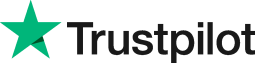How to work out percentage difference in Excel
• Home
• Courses
• Promotions
• Schedule
• Formats
• Our Clients

Forum home » Topics » How to work out percentage difference in Excel

# How to work out percentage difference in Excel

Having the ability to calculate the percentage difference between two figures in Excel allows you to effectively report on trends.

In the Finance arena, you would use it to see percentage difference from last year to the current year. In the HR arena, they may use this formula to work out the percentage difference between how many women and men were hired this year compared to the previous year.

To work out the percentage difference between one year and the next, you can use the following formula:

=(New Value - Old Value)/Old Value

=(B2-A2)/A2

Cell B2 contains the figure for 2000 which is 175000
Cell A2 contains the figure for 1999 which is 100000

So the percentage increase from 1999 to 2000 would be:

=(175000-100000)/100000 = 0.75 or a 75% increase

Now format the result as a percentage.

# Related forum posts:

## Percentage difference

Hi How would you work out the percentage difference between two cells (for example A1 and B1)? Is there a pre-programmed formula for this? Many thanks Anne

# Related articles

## Excel Course Tips and Tricks to Liven Up Your Excel Spreadsheets

 Welcome. Please choose your application (eg. Excel) and then post your question. Our Microsoft Qualified trainers will then respond within 24 hours (working days). Frequently Asked Questions What does 'Resolved' mean? Any suggestions, questions or comments? Please post in the Improve the forum thread.TrustScore 4.8 / 5       1238 reviews